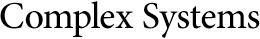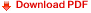## Undecidable Problems in Fractal GeometrySimant Dube
Department of Mathematics, Statistics, and Computing Science,
University of New England, Armidale NSW 2351, Australia

#### Abstract

In this paper, a relationship between the classical theory of computation and fractal geometry is established. Iterated Function Systems are used as tools to define fractals. It is shown that two questions about Iterated Function Systems are undecidable: to test if the attractor of a given Iterated Function System and a given line segment intersect and to test if a given Iterated Function System is totally disconnected. The proofs are very simple and are obtained by reducing the Post Correspondence Problem and by interpreting strings as numbers and concatenation operations as compositions of affine transformations. These results show that for every Turing machine there exists a fractal set which can be viewed, in a certain sense, as geometrically encoding the complement of the language accepted by the machine. One can build a fractal-based geometrical model of computation which is computationally universal.